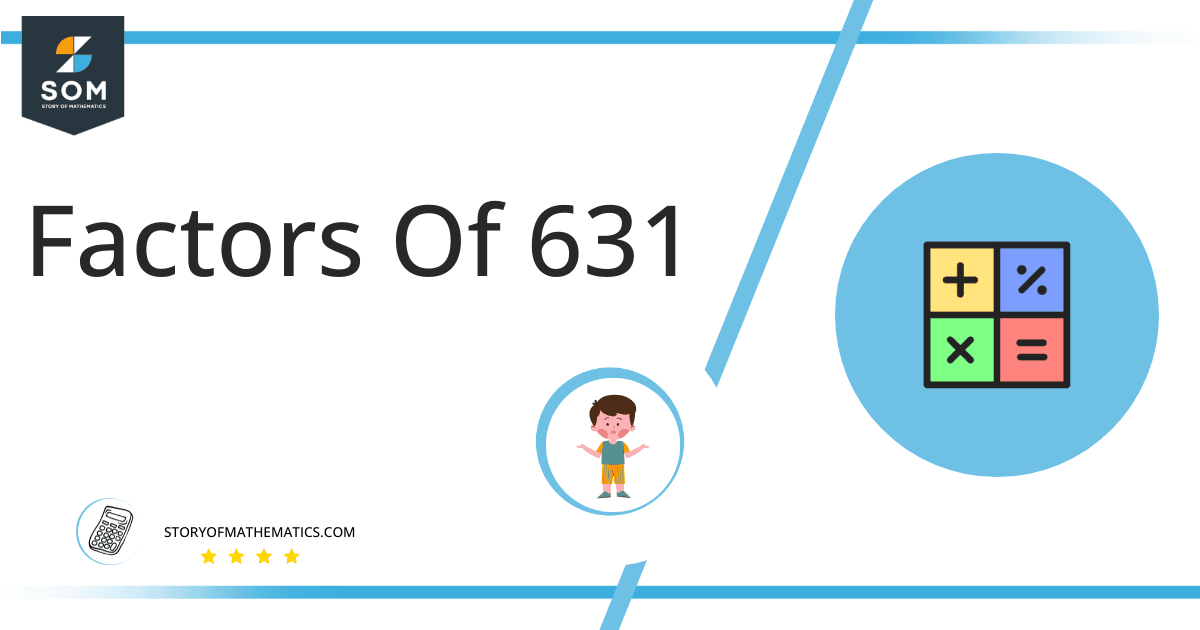# Factors of 631: Prime Factorization, Methods, and Examples

The factors of 631 are numbers that fully divide 631 into equal parts. Since 631 is a prime number therefore it cannot be divided by any other number except for 1 and the number 631 itself.Let us find out more about the number 631.

### Factors of 631

Here are the factors of number 631.

Factors of 631: 1 and 631

### Negative Factors of 631

The negative factors of 631 are similar to their positive aspects, just with a negative sign.

Negative Factors of 631: -1 and -631

### Prime Factorization of 631

The prime factorization of 631 is the way of expressing its prime factors in the product form.

Prime Factorization: 1 x 631

In this article, we will learn about the factors of 631 and how to find them using various techniques such as upside-down division, prime factorization, and factor tree.

## What Are the Factors of 631?

The factors of 631 are 1 and 631. These numbers are the factors as they do not leave any remainder when divided by 631.

The factors of 631 are classified as prime numbers and composite numbers. The prime factors of the number 631 can be determined using the prime factorization technique.

## How To Find the Factors of 631?

You can find the factors of 631 by using the rules of divisibility. The divisibility rule states that any number, when divided by any other natural number, is said to be divisible by the number if the quotient is the whole number and the resulting remainder is zero.

To find the factors of 631, create a list containing the numbers that are exactly divisible by 631 with zero remainders. One important thing to note is that 1 and 631 are 631’s factors as every natural number has 1 and the number itself as its factor.

1 is also called the universal factor of every number. The factors of 631 are determined as follows:

$\dfrac{631}{1} = X$

Therefore, 1 and 631 are the factors of 631.

### Total Number of Factors of 631

For 631, there are two positive factors and two negative ones. So in total, there are four factors of 631.

To find the total number of factors of the given number, follow the procedure mentioned below:

1. Find the factorization/prime factorization of the given number.
2. Demonstrate the prime factorization of the number in the form of exponent form.
3. Add 1 to each of the exponents of the prime factor.
4. Now, multiply the resulting exponents together. This obtained product is equivalent to the total number of factors of the given number.

By following this procedure, the total number of factors of 631 is given as:

Factorization of 631 is 1 x 631.

The exponent of 1 and 631 is 1.

Adding 1 to each and multiplying them together results in four.

Therefore, the total number of factors of 631 is four. Two are positive, and two factors are negative.

### Important Notes

Here are some essential points that must be considered while finding the factors of any given number:

• The factor of any given number must be a whole number.
• The factors of the number cannot be in the form of decimals or fractions.
• Factors can be positive as well as negative.
• Negative factors are the additive inverse of the positive factors of a given number.
• The factor of a number cannot be greater than that number.
• Every even number has 2 as its prime factor, the smallest prime factor.

## Factors of 631 by Prime Factorization

The number 631 is a prime number. Prime factorization is a valuable technique for finding the number’s prime factors and expressing the number as the product of its prime factors.Before finding the factors of 631 using prime factorization, let us find out what prime factors are. Prime factors are the factors of any given number that are only divisible by 1 and themselves.

To start the prime factorization of 631, start dividing by its most minor prime factor. First, determine that the given number is either even or odd. If it is an even number, then 2 will be the smallest prime factor.

Continue splitting the quotient obtained until 1 is received as the quotient. The prime factorization of 631 can be expressed as:

631 = 1 x 631

## Factors of 631 in Pairs

The factor pairs are the duplet of numbers that, when multiplied together, result in the factorized number. Factor pairs can be more than one depending on the total number of factors given.For 631, the factor pairs can be found as:

1 x 631 = 631

The possible factor pairs of 631 are given as (1, 631).

All these numbers in pairs, when multiplied, give 631 as the product.

The negative factor pairs of 631 are given as:

-1 x -631 = 631

It is important to note that in negative factor pairs, the minus sign has been multiplied by the minus sign, due to which the resulting product is the original positive number. Therefore, -1 and -631 are called negative factors of 631.

The list of all the factors of 631, including positive as well as negative numbers, is given below.

Factor list of 631: 1, -1, 631, and -631

## Factors of 631 Solved Examples

To better understand the concept of factors, let’s solve some examples.

### Example 1

How many factors of 631 are there?

### Solution

The total number of Factors of 631 is two.

Factors of 631 are 1 and 631.

### Example 2

Find the factors of 631 using prime factorization.

### Solution

The prime factorization of 631 is given as:

631 $\div$ 631 = 1

So the prime factorization of 631 can be written as:

1 x 631 = 631